# MCAT Physical : Atomic Nucleus

## Example Questions

2 Next →

### Example Question #11 : Atomic Nucleus

Nuclear attraction is a force between which two subatomic particles?

Neutrons and protons

Neutrons and electrons

Neutrons and neutrons

Protons and electrons

Neutrons and protons

Explanation:

Nuclear attraction is a force that holds together the molecules in the nucleus of an atom. Remember that there are a total of three subatomic particles in an atom: protons, neutrons, and electrons. Protons are positively charged, neutrons are neutral, and electrons are negatively charged. Of the three subatomic particles, only protons and neutrons are found inside the nucleus. Nuclear attraction must occur between a neutron and a proton. This force counteracts repulsion between protons to hold the nucleus together. Electrons are found in electron shells outside the nucleus and do not participate in nuclear attraction.

### Example Question #11 : Nuclear Chemistry And Electrons

Which of the following is true about nuclear attraction force?

I. It occurs between two quarks

II. It occurs between two hadrons

III. It is classified as a strong force

II only

I and II

I and III

I only

II only

Explanation:

Nuclear forces occur between protons and neutrons in the nucleus of an atom. Recall that quarks are elementary particles that make up composite particles called hadrons. Protons and neutrons are types of hadrons; therefore, nuclear forces occur between two hadrons.

There are four major types of fundamental forces: gravitational force, electromagnetic force, weak force, and strong force. Gravitational force is the attractive force between two bodies, electromagnetic force is a combination of the force between charges and magnetic force, weak force is the force between a W boson and a Z boson, and strong force is the force that occurs between two quarks. Nuclear forces between protons and neutrons are an effect created by the strong force between two quarks; however, nuclear forces are not classified as a type of strong force.

### Example Question #12 : Atomic Nucleus

Choose the best answer for the following question.

You have a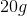sample of an unknown substance with a half-life of 3 days. After 9 days how much of the substance remains?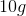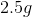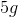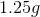Explanation:

Since the half-life of the sample is 3 days long, throughout the course of 9 days, the sample will undergo a half-life cycle 3 times. This means we have to half the mass of the original sample 3 times.

We originally start withAfter 3 days:After 6 days:After 9 days:Another way to solve these types of problems is using this formula: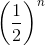whereis the number of half lives.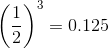thus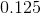or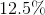of the original sample remains.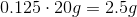Therefore after 9 days, there will beof the original sample remaining.

2 Next →

### All MCAT Physical Resources